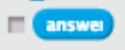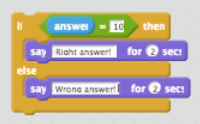Saturday, July 25, 2015

A Simple Math Game in Scratch - Introducing Conditionals & Comparators

Video games are ever popular among kids. And there are plenty of Math tutorials out there that use the format of video games to entice kids to practice their arithmetic. My child definitely enjoys working with these, and I am sure yours do as well. So, how about getting the kids to design and program their own little Math video game?

The students shall design the Math questions (and figure out the answers) to use in the game. They shall use comparators to decide if the answers entered by the user are correct or not. Variables shall be used to keep track of the number of right and wrong answers. This could potentially be a project for grade 4 and above, since variables are introduced as part of grade 4 Math.

Aim:

Design a simple, interactive Math Video Game, with a pre-decided set of questions. The user gets to enter the answers, and the game should tell the user if the answers are right or wrong. The game should also keep track of the number of right and wrong answers, and display the scores at the end of the game.

Programming Concepts Introduced:

1. Conditionals    (If - Then - Else)
2. Comparators   (Equality testing)
3. Variables        (To keep track of the game scores)

Implementation:

Here's the Scratch project that my child and I made:  A Simple Math Game.

The Design Process:

The exercise is intended to introduce the students to Conditionals and Comparators.

To make the game, start off by writing up a few Math questions and their answers. Have this list ready with you. Next, choose a fun sprite and a background, to make the game look interesting. Now, build up the program step-by-step.Look at the requirements for the assignment. The game asks questions to the user and the user types in answers. The sprite could ask the questions. Since we need to keep track of the user input, the best option is to use the "ask and wait" instruction in the "Sensing" area of the Scratch Instruction Set.Once the user types in an answer, it gets stored in the "answer" variable, again available in the "Sensing" area of the Scratch Instruction Set. This is the value that we need to compare against the pre-set correct answers from our list.

Introduce Comparators:  Equality operator

Lead the discussion with the question "How can we find out if the user entered the right answer?"In the "Operators" area of the Scratch Instruction set, you will find 3 different comparators - less than, equal to & greater than. Discuss with the kids as to which one of these would be the best choice for checking if the user entered the right answer (we shall use the "equal to" operator in this case to compare the user input to the right answer).

For example, if the right answer is 10, we shall check the user input using the following comparator:

Introduce Conditionals:  If-Then-ElseThe next step is to decide what to do if the answer is right or wrong. Here's where the If-Then-Else statement comes into play. If-Then-Else can be found in the "Control" section of the Scratch Instruction Set. You could start by giving the students a few simple examples:
1.  If we behave well, then the teacher will give us extra recess time;
else we shall have a shorter recess.
2.  If it rains, I shall bring my umbrella to school;
else I shall leave my umbrella at home.

The simplest step could be the sprite announcing that the answer is right or wrong:
• Every time the user's answer is correct, say "Right Answer" &Use of Variables:

The final requirement for the game is to keep track of the number of right and wrong answers. We need two variables for this purpose. The variables can be created under the "Data" section:  Click on "Make a Variable", and choose a (meaningful) name for the variable. Make two variables, say "Points" & "Wrong Answers", as in the sample project. Make sure to display both variables on screen, using the "Show Variable" instruction.

Initialize both variables to zero at the start of the game. Discuss with the students why we need to do so. Now, add the following steps to the above If-Then-Else statement:

• Every time the user's answer is correct, increase the variable "Points" &
• Every time the answer is wrong, increase the variable "Wrong Answers".

We are almost done with the design at this point. To start and stop the game, use an event, such as "When green flag clicked" or a key press, etc., found under the "Events" section. You can add in as many questions as you like to make the game interesting. Make sure to test the code every few steps, as you build up the program.

Note:

If you look at the sample project that my child and I made, you would see that we grouped the questions into different blocks/function. This keeps the code clean and provides for easier maintenance and testing, but it's definitely not a requirement for creating the game.

Have fun creating your own Math Video Game!!